# Algebra II : Solving and Graphing Radicals

## Example Questions

1 2 5 6 7 8 9 10 11 13 Next →

### Example Question #8 : Graph Square Root, Cube Root, And Piecewise Functions

Which of the following is the graph of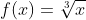?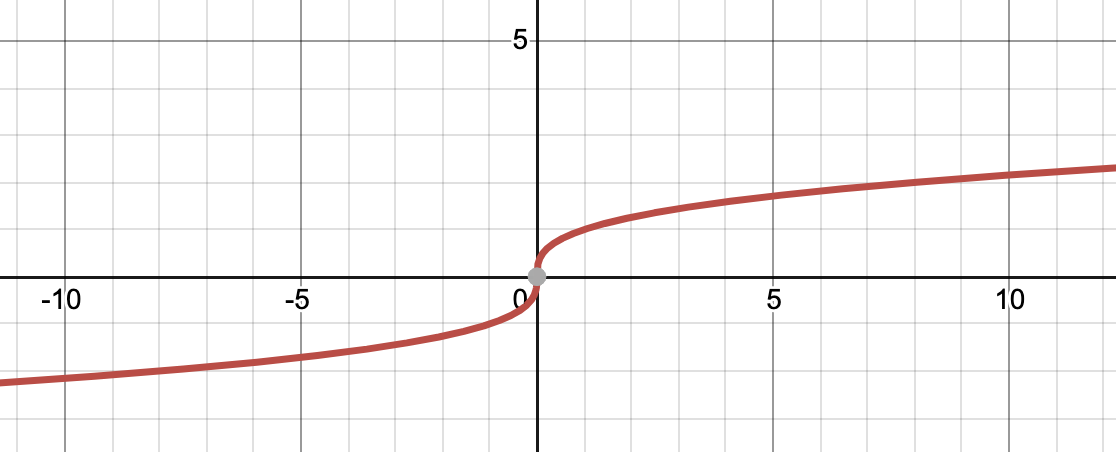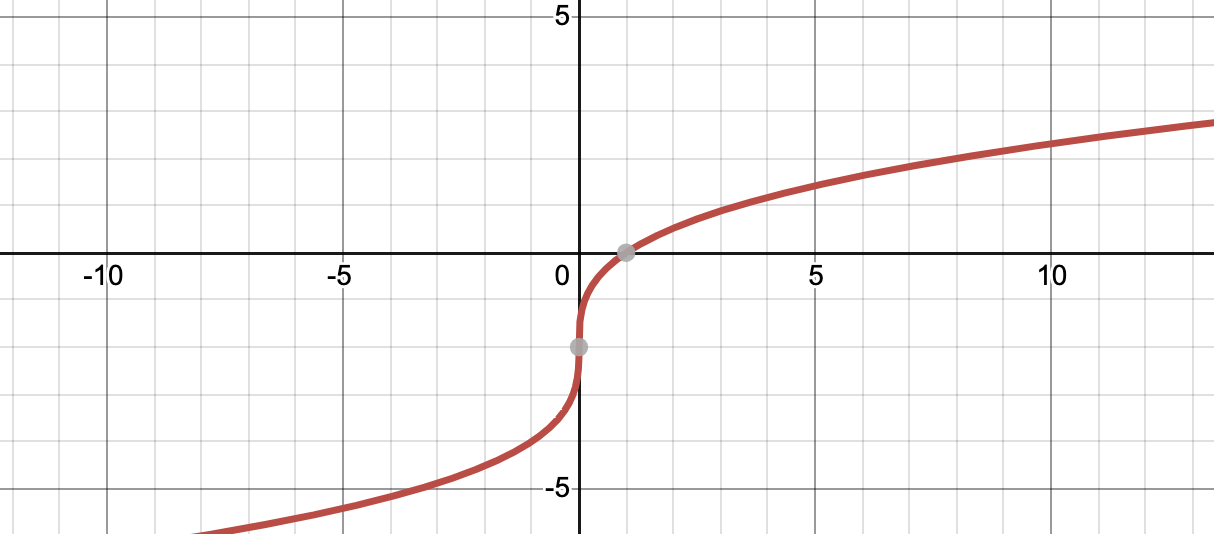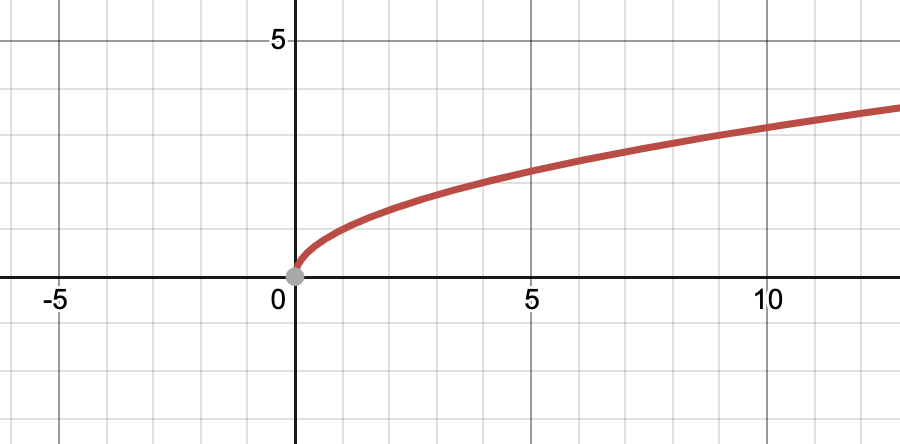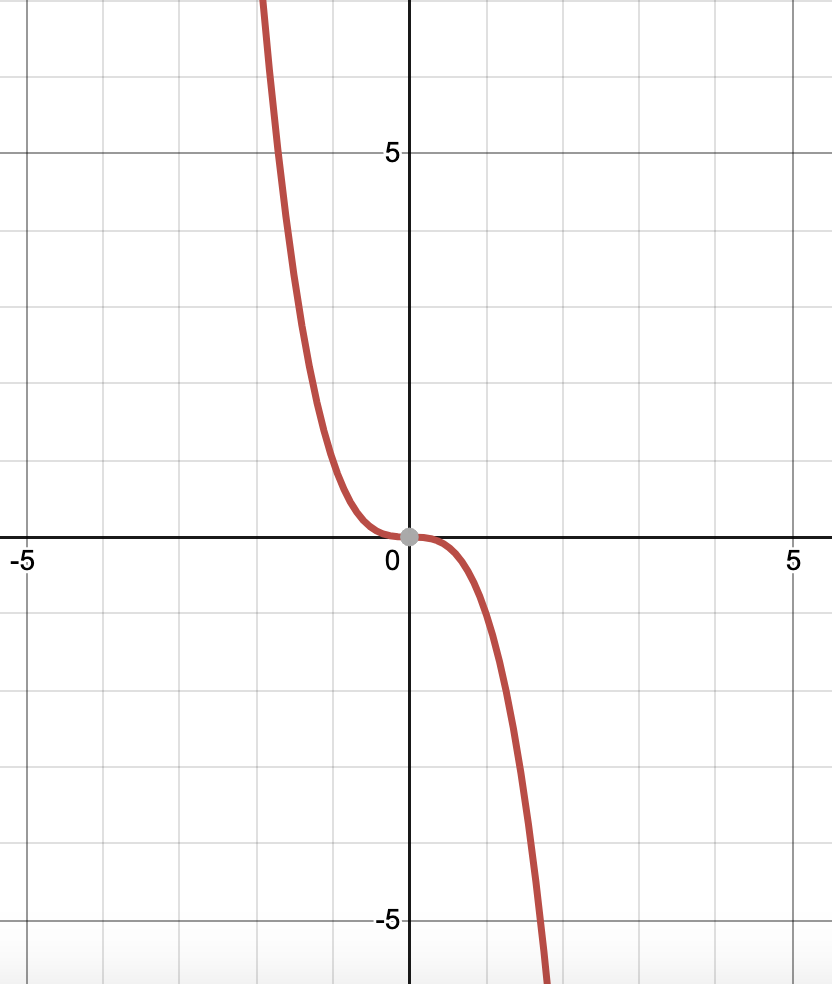Explanation:

The graph ofis.

The other graphs shown are those of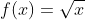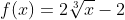, and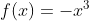.

### Example Question #9 : Graph Square Root, Cube Root, And Piecewise Functions

Which of the following is the graph of?Explanation:

The graph ofisThe other graphs pictured are, and.

### Example Question #10 : Graph Square Root, Cube Root, And Piecewise Functions

State the x-intercept, y-intercept, domain, and range of the function, pictured below.x-intercept: none

y-intercept: none

Domain: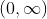Range:x-intercept: none

y-intercept: none

Domain: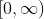Range:x-intercept: x=0

y-intercept: y=0

Domain:Range:x-intercept: x=0

y-intercept: y=0

Domain:Range:x-intercept: x=0

y-intercept: y=0

Domain:Range:Explanation:

This graph intersects the x axis only once and the y axis only once, and these happen to coincide at the point (0,0). Therefore the x-intercept is at x=0 and the y-intercept is at y=0. The graph will go on infinitely to the right. However, the leftmost point is (0,0.) Likewise, the graph will go infinitely high, but its lowest point is also (0,0). Therefore, its domain isand its range is also.

### Example Question #11 : Graph Square Root, Cube Root, And Piecewise Functions

State the x-intercept, y-intercept, domain, and range of the function, pictured below.x-intercept: x=10

y-intercept: y=3

Domain: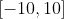Range: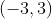x-intercept: x=10

y-intercept: y=3

Domain: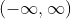Range:x-intercept: x=0

y-intercept: y=0

Domain:Range:x-intercept: x=0

y-intercept: y=0

Domain:Range:x-intercept: x=0

y-intercept: y=0

Domain:Range:Explanation:

This graph crosses the x axis only once and the y axis only once, and these happen to coincide at the point (0,0). Therefore the x-intercept is at x=0 and the y-intercept is at y=0. The graph will go on infinitely to both the left and the right. Additionally (and despite the fact that it won't happen as quickly), the graph also will go infinitely high in both its positive and negative y values. Therefore, both the domain and range of this graph are.

1 2 5 6 7 8 9 10 11 13 Next →

### All Algebra II Resources